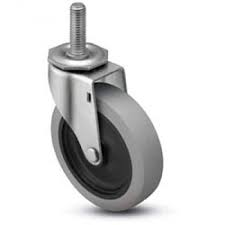# Rotate child object opposite to moving parent object's direction

I have a table object with wheels like this:When I move the table forward, these wheels point away from the direction of the table. That is, it rotates away from the direction of the table. I’ve tried implementing this behavior in Unity with the code below:

``````public class WheelOne : MonoBehaviour
{
[SerializeField] private GameObject wheelTableObj;
[SerializeField] private float rotateSpeed = 1f;
private Vector3 previousPos;
private Vector3 currentPos;

void Update()
{
if (wheelTableObj.GetComponent<Rigidbody>().velocity != Vector3.zero) {
currentPos = wheelTableObj.transform.position;

// Direction wheelTableObject is moving
Vector3 direction = (currentPos - previousPos).normalized;

//Direction wheel should be facing
Vector3 oppositeDirection = -direction;

transform.forward = Vector3.Slerp(transform.forward, oppositeDirection, Time.deltaTime * rotateSpeed);

}
}

void Start() {
if (wheelTableObj.GetComponent<Rigidbody>().velocity != Vector3.zero) {
previousPos = wheelTableObj.transform.position;
}
}
}
``````

The direction of the parent table is calculated by subtracting the current position and the previous position of the table. Inverting this direction gives me the direction the child wheel should be facing. Using Vector3 slerp allows me to rotate the wheel over time. However I got weird results where it would correctly rotate instantly when I pushed the table in one axis, but when I continue to push it in another axis, its rotation keeps getting slower and slower. I’ve made the rotateSpeed a controllable value but it doesn’t seem to change anything. Any help would be appreciated!